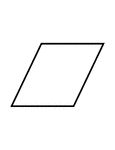### Flashcard of a Parallelogram

A flashcard featuring an illustration of a Parallelogram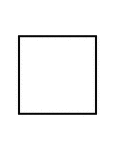### Flashcard of a polygon with four equal sides

A flashcard featuring an illustration of a polygon with four equal sides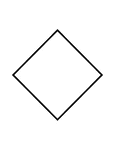### Flashcard of a polygon with four equal sides

A flashcard featuring an illustration of a polygon with four equal sides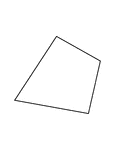### Flashcard of a polygon with four unequal sides

A flashcard featuring an illustration of a polygon with four unequal sides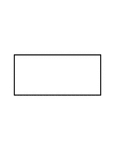### Flashcard of a Rectangle

A flashcard featuring an illustration of a Rectangle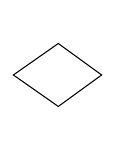### Flashcard of a Rhombus

A flashcard featuring an illustration of a Rhombus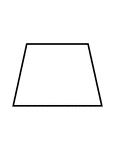### Flashcard of a Trapezoid

A flashcard featuring an illustration of a Trapezoid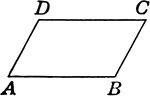### Parallelogram

Parallelogram with angles labeled.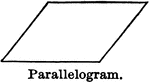### Parallelogram

Illustration of a parallelogram, a quadrilateral which has opposite sides parallel.### Parallelogram With Vertices Labeled

An illustration of a parallelogram with vertices labeled.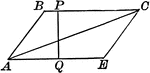### Altitude and Diagonal of a Parallelogram

Illustration of a parallelogram with altitude and diagonal drawn.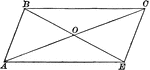### Parallelogram With Diagonals Bisecting Each Other

Illustration of a parallelogram with diagonals drawn to show they bisect each other.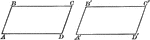### Equal Parallelograms

Illustration of a two equal parallelograms. Two parallelograms are equal, if two sides and the included…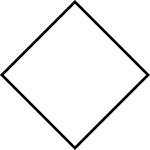### 4-sided Polygon

Polygon consisting of 4 sides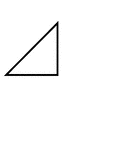### Fractions of 4-sided Polygon

1/4 of a 4 sided polygon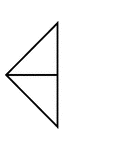### Fractions of 4-sided Polygon

2/4 of a 4 sided polygon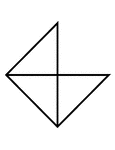### Fractions of 4-sided Polygon

3/4 of a 4 sided polygon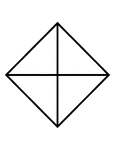### Fractions of 4-sided Polygon

4/4 of a 4 sided polygon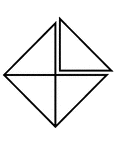### Fractions of 4-sided Polygon

4/4 of a 4 sided polygon with one piece detached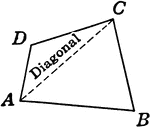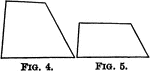### Equiangular Polygons

Illustration of a two polygons. Polygons may be mutually equiangular without being mutually equilateral.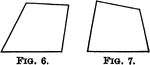### Equilateral Polygons

Illustration of two polygons. Polygons may be mutually equilateral without being mutually equiangular…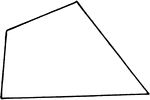Illustration of a quadrilateral, a four-sided figure.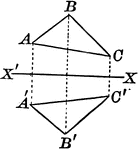### Axis of Symmetry Drawn on a Quadrilateral

Illustration of an axis of symmetry drawn with respect to a quadrilateral.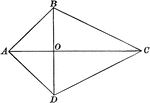Illustration of a quadrilateral which has two adjacent sides equal and the other two sides equal. It…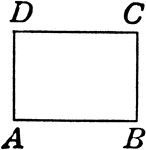### Rectangle

Rectangle with angles labeled. Parallelogram with right angles.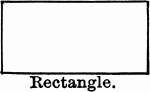### Rectangle

Illustration of a rectangle, which is a parallelogram with all angles right angles.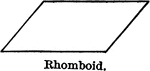### Rhomboid

Illustration of a rhomboid, which is a parallelogram which has its angles oblique angles.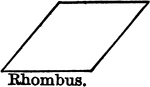### Rhombus

Illustration of a rhombus, which is a rhomboid with all sides equal.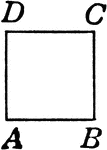### Square

Square with angles labeled. A rectangle with four equal sides.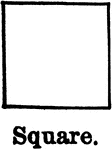### Square

Illustration of a square, a rectangle with all sides equal.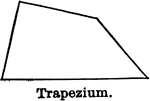### Trapezium

Illustration of a trapezium, a quadrilateral which has no two sides parallel.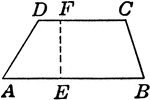### Trapezoid

Trapezoid with angles and height labeled.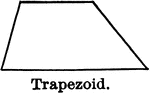### Trapezoid

Illustration of a trapezoid, a quadrilateral which has two and only two sides parallel.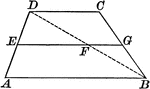### Trapezoid With Median

Illustration of a trapezoid, with the median drawn. The median of a trapezoid is parallel to the bases,…